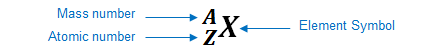# Problem: 41Ca decays by electron capture. The product of this reaction undergoes alpha decay. What is the product of this second decay reaction?a. Potassium (K)b. Scandium (Sc)c. Chlorine (Cl)d. Rubidium (Rb)e. Vanadium (V)

###### FREE Expert Solution

We’re being asked to determine the product of the second decay reaction when 41Ca decays by electron capture and the product of this reaction undergoes alpha decay

The element can be represented as:• 1st Reaction: Electron capture

occurs when a nucleus absorbs an electron to create a new element
an electron has no atomic mass and is represented by e

99% (313 ratings)###### Problem Details

41Ca decays by electron capture. The product of this reaction undergoes alpha decay. What is the product of this second decay reaction?

a. Potassium (K)
b. Scandium (Sc)
c. Chlorine (Cl)
d. Rubidium (Rb)